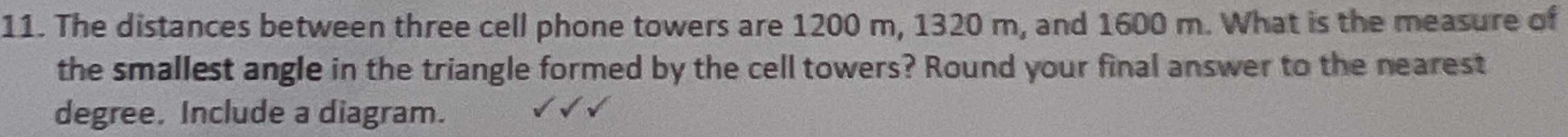### Still have math questions?

Trigonometry
QuestionThe distances between three cell phone towers are $$1200 m , 1320 m$$ , and $$1600 m$$ . What is the measure of the smallest angle in the triangle formed by the cell towers?

smallest angle = 47$$°$$